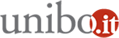# Statistics seminar: "Re-estimation of the general linear model after adding and deleting"

Seminario di Statistica

01/10/2013 dalle 11:30 alle 14:00

Dove Dipartimento di Scienze Statistiche - Via Belle Arti 41 - Aula IVAggiungi l'evento al calendario

Authors: Erricos Kontoghiorghes
Cyprus University of Technology, Cyprus
Email:  erricos@cut.ac.cy

Abstract
:
It is often computationally infeasible to re-estimate afresh a large-scale model when a small number of observations is sequentially modified. Furthermore, in some cases a dataset is too large and might not be able to fit in a computer's memory and in such cases out of core algorithms need to be developed. Similarly data might not be available at once and recursive estimation strategies need to be developed. Within this context the aim is to design computationally efficient and numerically stable algorithms. Initially, the re-estimation of the generalized least squares (GLS) solution after observations are deleted, known as downdating, is examined. The problem of deleting observations from the general linear model (GLM) with a possible singular dispersion matrix is considered. The new method to estimate the downdated-GLM, updates the original GLM model with the imaginary deleted observations. This results to a non-positive definite dispersion matrix which comprises complex covariance values. This updated-GLM with imaginary values has been proven to derive the same GLS estimator as that of solving afresh the original GLM after downdating. The estimation of the downdated-GLM is formulated as a generalized linear least squares problem (GLLSP). The solution of the GLLSP derives the GLS estimator. The main computational tool is the generalized QR decomposition which is employed based on hyperbolic Householder transformations, however, no complex arithmetic is used in practice. The special case of computing the GLS estimator of the downdated-SUR (seemingly unrelated regressions) model is considered. The special structure of the matrices and properties of the SUR model are efficiently exploited in order to reduce the computational burden of the estimation algorithm. Furthermore, a recursive estimation of the simultaneous equations model is proposed. That is, a new strategy to compute recursively the three stage least squares estimator is developed. The method is extended to the problem of concurrently adding and deleting observations from the model.